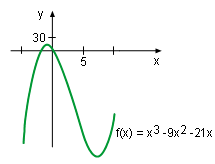Ch 4. The Mean Value Theorem Multimedia Engineering Math Maximum & Minimum Rolle'sTheorem Mean ValueTheorem MonotonicFunctions First DerivativeTest Concavity &Inflection SecondDerivative Test
 Chapter 1. Limits 2. Derivatives I 3. Derivatives II 4. Mean Value 5. Curve Sketching 6. Integrals 7. Inverse Functions 8. Integration Tech. 9. Integrate App. 10. Parametric Eqs. 11. Polar Coord. 12. Series Appendix Basic Math Units Search eBooks Dynamics Fluids Math Mechanics Statics Thermodynamics Author(s): Hengzhong Wen Chean Chin Ngo Meirong Huang Kurt Gramoll ©Kurt GramollMATHEMATICS - THEORY In this section, the second derivative test is introduced along with an application.Function f(x) = x3 - 9x2 - 21x Diagram In order to find the maximum and minimum points of a function, the critical points of the function need to be calculated first. However, it is unsure whether the function value is maximum or minimum at these specific point. It is easy to understand this by finding the minimum value of function f(x) = x3 - 9x2 - 21x in the range between -5 and 10. The minimum value of the function occurs at the critical points where the function's derivative is 0 or does not exist. Calculating the derivative of f(x) gives      df(x)/dx = d(x3 - 9x2 - 21x)/dx                  = (d(x3 ) - d(9x2) - d(21x))/dx                  = 3x2 - 18x - 21                  = 3(x - 7)(x + 1) When df(x)/dx = 0      3(x - 7)(x + 1) = 0 So x = 7 x = -1 Thus, the critical point is at x = 7 and x = -1 because both points are in (-5, 10). Although the local extreme value occurs at x = 7 or x = -1, it is not sure that which one is the maximum and which one is the minimum. According to Function f(x) = x3 - 9x2 - 21x Diagram when x = 7, the function value is minimum, and when x = -1 the function value is maximum. Is there a method to justify the maximum and minimum value point other than plotting the function's diagram or calculating the function's value? The answer is yes and is discussed below. The Second Derivative Test The Second Derivative Test states: Suppose the second derivative of a function is continuous on (a, b) and c is in this interval. If df(c)dx = 0 and df2(c)/dx2 > 0, then the function has a local minimum at c. If df(c)dx = 0 and df2(c)/dx2 < 0, then the function has a local maximum at c. The second derivative test can be better understood by continuing the previous example. In the previous example, the critical points is found when the derivative is 0, and this satisfies the condition of the second derivative test, df(c)dx = 0. In other words, the value of the first derivative of the function at 7 and -1 is 0. In order to use second derivative test, the second derivatives at x = 7 and x = -1 are needed. Take another derivative to the first derivative equation, df(x)/dx = 3x2 - 18x - 24, to obtain the second derivative. That is      df2(x)/dx2 = df(df(x)/dx))/dx                    = df(3x2 - 18x - 24)/dx                    = d(3x2)/dx - d(18x)/dx - d(24)/dx                    = 6x -18 When x = 7      df2(x)/dx2 = 6x -18 = 24 > 0 So the function has a local minimum at this critical point. When x = -1      df2(x)/dx2 = 6x -18 = -24 < 0 So the function has a local maximum at this critical point. This result is confirmed by the Function f(x) = x3 - 9x2 - 24x Diagram.